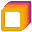samuel bancroft

@samuelbancroft

Keep reading, keep studying, the more you learn the more you change. If you are doing the Python lessons please join this discord channel https://discord.gg/kugXx9KY but please follow the rules

Promethea

discord.gg/XZQwPwaS97

389

Followers

0

Following

Joined Mar 23, 2021As you can see by the image, we are continuing with "if" statement, but this time it works different. The first "if" statement in this case is false, then thats when we get to the elif, this is another if statement just inside that if statement, if one of the elif's are true then it will stop there, execute the code insde the scope of that if statement then move on the next section of code

When it comes to elif's thet only go after a if statement, and never before there will be a error if that was to happen.

Dont forget to join the discord channel if you need help

https://discord.gg/XZQwPwaS97

💻

Learning Python

🚶 On a walk

1 IDEAAs you can see in this image i am using variables this time in the if statement. As was mentioned in the first part about if statements that they only return True or False. I have 2 if statements but only one is printing out to the console, this is because only one of them is true, join the dicsord channel and let me know why the second is not printing.

If statements can be used for alot of different situations, for example from simple text based games, to data processing to AI, IF statements are fundemental in python.

Dont forget to join discord if you need help

https://discord.gg/XZQwPwaS97As you can see in the image, we are working with conditional if statements. This is used to find out if something is True or False. As you can see i used '<', this means less than, so in this statement i am asking is '1 less than 10' and if true it will run the print inside. There is one key difference here than normal prints that we have done and that is the indent, this is saying if the if statement is True then go in to the scope, the indent and run all the code in there then move on.

Next part more on if statements.

Dont for get to join discord for help

https://discord.gg/XZQwPwaS97So as you can see from the image we are using 2 variables, x and y. Like in the previous lessons explained about variables, x contains the string Hello World, what makes it a string is " " the use of these quotation marks. Next in y there is a integer value 10. Then i am adding them both together, but as you can see it has given a error when i tried to print it. This is because you cant use mathmatical operators when you have one string and one number, you can add string and string but this is for another lesson.

Dont forget to jon the Discord channel for help.

https://discord.gg/vaQkyxZPE5For todays part we, are looking at basic numerical operators. In the image i have created 2 integer variables and then in the print i have used both variables and then used the operator inbetween them, so to add them we use '+', to subtract '-', mulyply '*' and finally divide '/'.

As you can see by the outputs it has worked, so 10 + 5 = 15, 10/5 = 2. These are basic operators for python, you can perform squaring and so on but thats for future content.

Next lesson will be about what happens if you added a string and integer together.

Dont forget to join Discord for help.

❤️ Brainstash Inc.